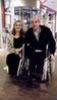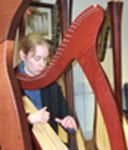## Hugging the world

Your platform. Get to know each other (but don't forget about us!)

Moderator: All Moderators

### Re: Hugging the world

Here, G!!!!!((((((((((((((((((((((G)))))))))))))))))))))))))))))))

I'll second G's comment though, where is everybody???? (((((((((((((((((((((((Forum)))))))))))))))))))))))))))
Dave

From Wales...via Birmingham!!!!Dab051176

Posts: 1299
Joined: Wed Mar 05, 2008 1:00 pm
Location: Flintshire, North Wales

### Re: Hugging the world

((((((((((((((((((((((Dave)))))))))))))))))))))))) for posting, and for listening to my olympic rambling so often lately! That deserves a doubleMusic and friendship - the answer to life's problems.

Whisper to the wild water
Step By Step
Slowly I turn - Máire BrennanGrania
Moderator

Posts: 11209
Joined: Tue Aug 31, 2004 2:26 pm
Location: England

### Re: Hugging the world

((((((((((((((((A_S, Grania, Dave))))))))))))))))))) I'm usually around, just not postingLuned

Posts: 1018
Joined: Sat Mar 24, 2007 5:19 pm
Location: Poland

### Re: Hugging the world

((((((((((Fories & Moya)))))))))) Hope you are still here, at least some of you...

Anyway, "dobar dan", namely, that is how the locals say "hello" here in Croatia! Our family holiday here in Dubrovnik is going well indeed, there is SO much to see and do here that I could easily write a book about it (or two!), but the heat (constantly around +30C) is slowing us down quite much. Well, at least it means that we have to keep this as a TRUE holiday week rather than running from place to place all the time... And, although I cannot swim due to my disability, I quite like it when I can lie down to one of the hammocks in the park of the hotel and just enjoy the sunshine! We do have one excursion to go to later this week though, we will visit the neighbouring country Montenegro so I am looking forward to it very much!

The price level is quite close to the euro level in Finland, but the currency being called the kuna (one euro is about 7 kunas). People do speak good English, are friendly and the general atmosphere is lovely indeed. The landscape is wonderful as well, mountains and hills but also lush parks and lots of flowers. Quite similar to what we experienced in Slovenia back in 2007. Obviously the heat has a downside as well, namely, we found a wonderful place near our hotel which makes pizzas in a big open-air oven which is warmed by burning logs inside it, but because of the heat, we cannot eat there as often as I would have wanted (LOVE their calzone!)! Well, luckily the ice-cream portions are HUGE, so that compensates a LOT! All in all, I can recommend this country wholeheartedly for anyone, especially Dubrovnik! I have seen people of all ages and many nationalities here so far...

Anyway, my online time is running short soon, so I must log off from here and surf somewhere else as well. All the very best for each and every forummer, and of course Moya (in case you have any time to log in here)!

Suvi

PS. I will try to upload some of our holiday photos to my Flickr account as soon as we return to Finland so you can see them as well!
"Your golden breath took darkness away, if I ask, You won't let me go..."cantilena91

Posts: 12992
Joined: Fri Sep 05, 2003 6:33 am
Location: city of Oulu, Finland

### Re: Hugging the world

(((((((((((((((((((((((Suvi))))))))))))))))))))))))))))))) Hope you had a great holiday in Croatia!!!!!

OK, folks..... One hug-fest coming right up........((((((((((((((((((G and Eirinn)))))))))))))))))) Fantastic to see you both in Gweedore the other weekend.......

((((((((((((((((((((Tim and Aisling))))))))))))))))))))))) For helping out musically!((((((((((((((((((((((((Cormac))))))))))))))))))))))) and of course................... ((((((((((((((((((((((((((((((((((((((((((((((Moya)))))))))))))))))))))))))))))))) Without whom we wouldn't have had the opportunity to have to such fun-filled weekends!!!!!Dave

From Wales...via Birmingham!!!!Dab051176

Posts: 1299
Joined: Wed Mar 05, 2008 1:00 pm
Location: Flintshire, North Wales

### Re: Hugging the world

Seconding all of the above! (except for the hug to myself - but ((((((((((((((Dave))))))))))))))) great to see you too!)

Amazing weekend - fingers crossed for next year!

All together now....

TEIDHIR ABHAILE RIU....!!!!
Music and friendship - the answer to life's problems.

Whisper to the wild water
Step By Step
Slowly I turn - Máire BrennanGrania
Moderator

Posts: 11209
Joined: Tue Aug 31, 2004 2:26 pm
Location: England

### Re: Hugging the world

(((((((((((((((((((((Luned)))))))))))))))))))))))))). Just noticed your posting tally - Welcome to the Addicted club!!!!!!!Dave

From Wales...via Birmingham!!!!Dab051176

Posts: 1299
Joined: Wed Mar 05, 2008 1:00 pm
Location: Flintshire, North Wales

### Re: Hugging the world

thanks! and back at you ((((((((((((((((((Dave))))))))))))))))))) So it's official, I'm addicted! And around such a date!Luned

Posts: 1018
Joined: Sat Mar 24, 2007 5:19 pm
Location: Poland

### Re: Hugging the world

Just wanting to offer a BIG ribcrusher for our 60-year-young lady-of-the-house, wher'ever you are:
((((((((((((((((((((((((((((((((((((((((((((((((((((((((((((((Moya)))))))))))))))))))))))))))))))))))))))))))))))))))))))))))))))))))

Suvi
"Your golden breath took darkness away, if I ask, You won't let me go..."cantilena91

Posts: 12992
Joined: Fri Sep 05, 2003 6:33 am
Location: city of Oulu, Finland

### Re: Hugging the world

Such a great craic again last night, so nice to have so many of us there!

(((((((((((((((((((((((((((((((((((((((((((((((((((((Moya & the Duggans))))))))))))))))))))))))))))))))))))))))))))))))))))))))))

Thanks SO much for providing us the second chance for the great concert, and the live chat with you was just the icing on the cake!

Suvi
"Your golden breath took darkness away, if I ask, You won't let me go..."cantilena91

Posts: 12992
Joined: Fri Sep 05, 2003 6:33 am
Location: city of Oulu, Finland

### Re: Hugging the world

{{{{{{{{{{Forum}}}}}}}}}} - so sorry I haven't been around for ageshope to be around more going forward but so busy, with Little One growing up (just over 7 months old now, where does the time go?) and all that ......If you don't know what eschatology is then don't worry; it's not the end of the world.
Purveyor of fine sarcasm since 1966.
Angoid

Posts: 3678
Joined: Tue Mar 02, 2004 10:08 pm
Location: In the cave

### Re: Hugging the world

(((((((((((((((((((((((((((((((((((((((((((((((((((((((((((ANGUS!!!!)))))))))))))))))))))))))))))))))))))))))))))))))))))))))))))))))))))))))))))

Really really good to see you! (good also to see SOMEONE posting here!!!!) Hope you and the family are well - how is the little one? Still in charge?Music and friendship - the answer to life's problems.

Whisper to the wild water
Step By Step
Slowly I turn - Máire BrennanGrania
Moderator

Posts: 11209
Joined: Tue Aug 31, 2004 2:26 pm
Location: England

### Re: Hugging the world

It is in the nature of Little Ones to take charge, G!
I've been meaning to get here, but life's been very busy (as you can imagine)
Must send you some recent pictures!

{{{{{{{{{{G}}}}}}}}}}
If you don't know what eschatology is then don't worry; it's not the end of the world.
Purveyor of fine sarcasm since 1966.
Angoid

Posts: 3678
Joined: Tue Mar 02, 2004 10:08 pm
Location: In the cave

### Re: Hugging the world

((((((((((((((((Angus))))))))))))))))))))))))) Great to see you around!!!!(((((((((((((((((((((G))))))))))))))))))))))) I'm still here - honest!!!!!Dave

From Wales...via Birmingham!!!!Dab051176

Posts: 1299
Joined: Wed Mar 05, 2008 1:00 pm
Location: Flintshire, North Wales

### Re: Hugging the world

{{{{{{{{{{Dave}}}}}}}}}}If you don't know what eschatology is then don't worry; it's not the end of the world.
Purveyor of fine sarcasm since 1966.
Angoid

Posts: 3678
Joined: Tue Mar 02, 2004 10:08 pm
Location: In the cave

PreviousNext